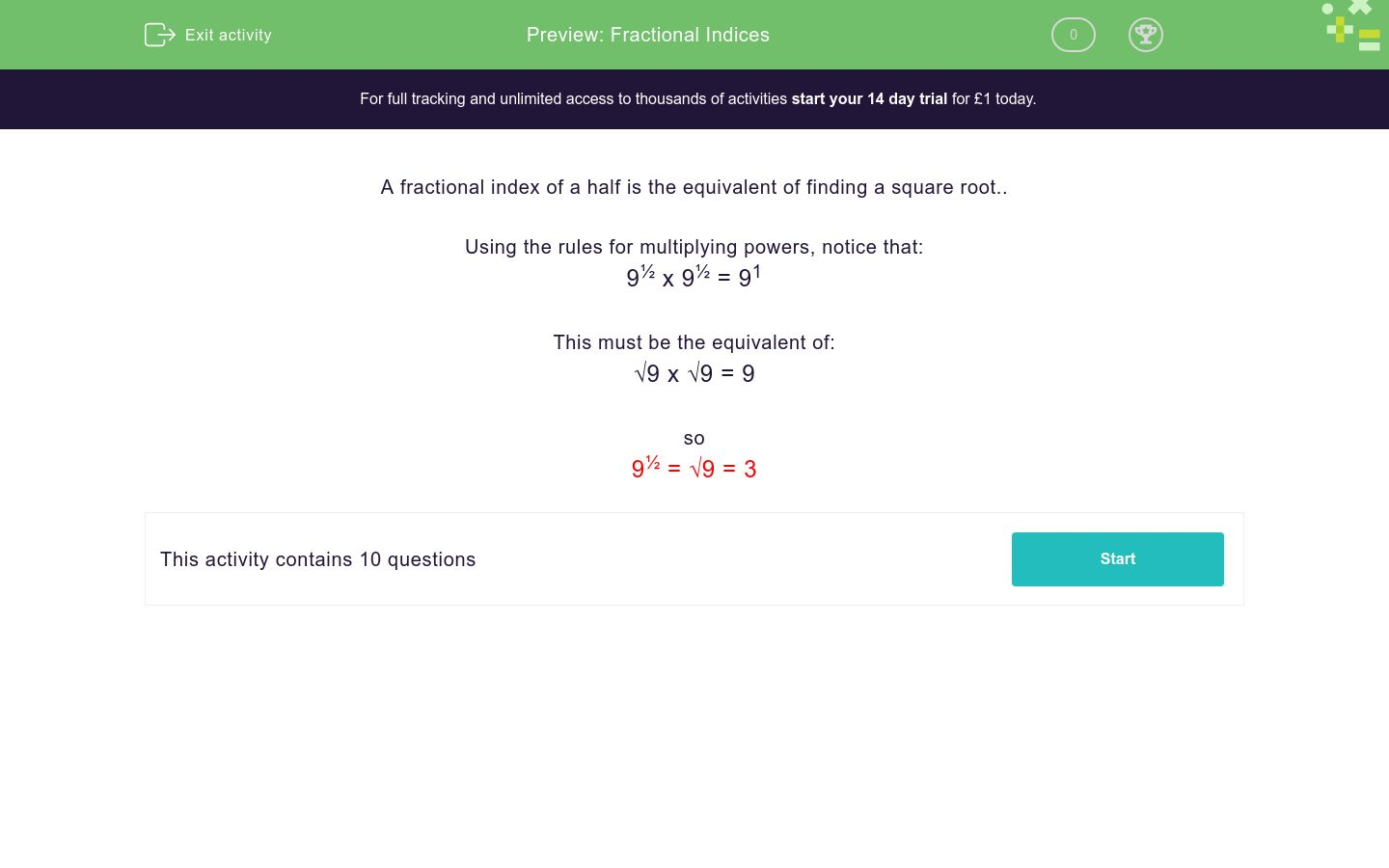# Fractional Indices

In this worksheet, students understand and use fractional indices.Key stage:  KS 4

Curriculum topic:  Number

Curriculum subtopic:  Calculate with Roots and Integer (Fractional) Indice

Difficulty level:### QUESTION 1 of 10

A fractional index of a half is the equivalent of finding a square root..

Using the rules for multiplying powers, notice that:

9½ x 9½ = 91

This must be the equivalent of:

√9 x √9 = 9

so

9½ = √9 = 3

Select the answer which is equal to:

16½

8

16½

4

Select the answer which is equal to:

36½

6

36½

18

Select the answer which is equal to:

1½

1

½

1.5

Select the answer which is equal to:

10½

5

√10

10.5

Select the answer which is equal to:

25½

25½

√12.5

√25

Select the answer which is equal to:

100½

10½

50

10

Select the answer which is equal to:

0½

½

0

1

Select the answer which is equal to:

10000½

10½

100

1000

Select the answer which is equal to:

1000½

√1000

100

33.3

Select the answer which is equal to:

16900½

√169

1300

130

• Question 1

Select the answer which is equal to:

16½

CORRECT ANSWER
4
• Question 2

Select the answer which is equal to:

36½

CORRECT ANSWER
6
• Question 3

Select the answer which is equal to:

1½

CORRECT ANSWER
1
• Question 4

Select the answer which is equal to:

10½

CORRECT ANSWER
√10
• Question 5

Select the answer which is equal to:

25½

CORRECT ANSWER
√25
• Question 6

Select the answer which is equal to:

100½

CORRECT ANSWER
10
• Question 7

Select the answer which is equal to:

0½

CORRECT ANSWER
0
• Question 8

Select the answer which is equal to:

10000½

CORRECT ANSWER
100
• Question 9

Select the answer which is equal to:

1000½

CORRECT ANSWER
√1000
• Question 10

Select the answer which is equal to:

16900½

CORRECT ANSWER
130
---- OR ----

Sign up for a £1 trial so you can track and measure your child's progress on this activity.

### What is EdPlace?

We're your National Curriculum aligned online education content provider helping each child succeed in English, maths and science from year 1 to GCSE. With an EdPlace account you’ll be able to track and measure progress, helping each child achieve their best. We build confidence and attainment by personalising each child’s learning at a level that suits them.

Get started#### Similar activities you may like

Start your £1 trial today.
Subscribe from £10/month.